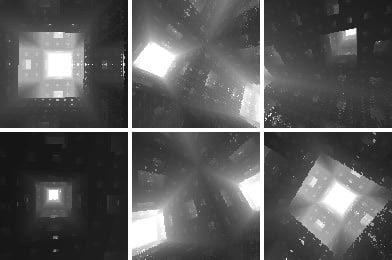# JSPONGY

319 words ~ 2-3 minsMathieu 'p01' Henri on October 23rd, 2009

# JSpongy

Since Mentor^TBC released Spongy, an amazing 128b intro raymarching a Menger sponge, I wondered how far such effect could be size optimized in JavaScript while keeping complex camera path. The answer: 281 bytes.The goal was to optimize for size and release the result as an intro in the demoscene spirit. That explains why although it is possible to cram a Menger Sponge raymarching in 284 bytes of HTML5 with a very scarce design, the release version fits in the 512b category and retain some of the style and complexity of the original.

## Menger sponge ?

The Menger sponge is a three-dimensional extension of the Cantor set and Sierpinski carpet first described by Austrian mathematician Karl Menger in 1926.

It starts as a single cube in which the central pillar is carved out in all three axis to make 20 smaller cubes on which the process is repeated.

## Source codes

### 511 bytes

``<body bgcolor=0 text=snow onload=N=[K=R.getContext('2d')];for(t=B=127,I=K.getImageData(0,0,q=64,q);t--;\$=Math.cos)N[t]=t/43&1;setInterval("t++;for(i=y=-1;y<1;y+=A)for(x=-1;x<1;x+=A=1/32,I.data[i+=4]=h+h)for(m=C=\$(a=t/86),S=\$(a+8),c=\$(b=t/B),s=\$(b+8),u=x*C+S,v=y*c-u*s,u=u*c+y*s,w=C-x*S,X=q+9*\$(a+b),Y=q+9*\$(b-a),Z=t,h=B;--h&&m<q;X+=u,Y+=v,Z+=w)for(m=1;N[X*m&B]+N[Y*m&B]+N[Z*m&B]<2&&m<q;m*=3);K.putImageData(I,0,0)",9)>JSpongy by p01<br><canvas id=R width=64 height=64 style=width:3in;height:3in;background:#fff>``

### 281 bytes

``<canvas id=R><svg onload=setInterval("for(i=q*q,C=M(t+=R.width=q),S=M(t+8);i--;R.getContext`2d`.fillRect(i/q,i%q,1,h/q))for(x=i%q/66-1,y=i/8712-1,m=w=x*C+S,X=Y=h=q,Z=t/q;m<--h;X+=y*C+w*S,Y+=y*S-w*C,Z+=C-x*S)for(m=1;(X*m%96&32)+(Y*m%96&32)+(Z*m%96&32)<64;)m*=3",M=Math.cos,t=q=132)>``

## Technical information

The camera travels in the fractal along a cycloid without ever touching it. The camera target rotates along the Y and Z axis.

The rendering itself is done using Canvas and ImageData by simply changing the Alpha channel of each pixel in order to gain a few bytes necessary to stay under 512b.

The Menger Sponge is raymarched at fixed steps for a maximum of 128 steps. Also the inside of the fractal is computed using the formula: `(Xm%3&1)+(Ym%3&1)+(Z*m%3&1)<2` where `m` represents the generation of the fractal.

The intrisinc resolution of the Canvas is 64 x 64.

## Karma

Of course Jspongy and Spongy are available on Pouet.net